Question

# If Pair is 104.6 kPa at 27.7 °C, what is Pair (in kPa) at a temperature...

If Pair is 104.6 kPa at 27.7 °C, what is Pair (in kPa) at a temperature of 37.5 °C?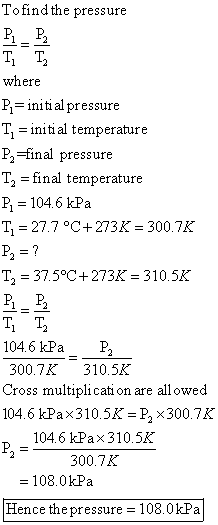#### Earn Coins

Coins can be redeemed for fabulous gifts.

Similar Homework Help Questions
• ### 7. An oxygen tank reads 130 kPa with a temperature of 25 °C. What will the...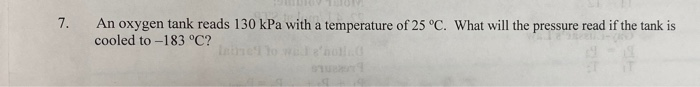7. An oxygen tank reads 130 kPa with a temperature of 25 °C. What will the pressure read if the tank is cooled to -183 °C?

• ### Resources Lx Give Up? A hot lump of 27.7 g of copper at an initial temperature...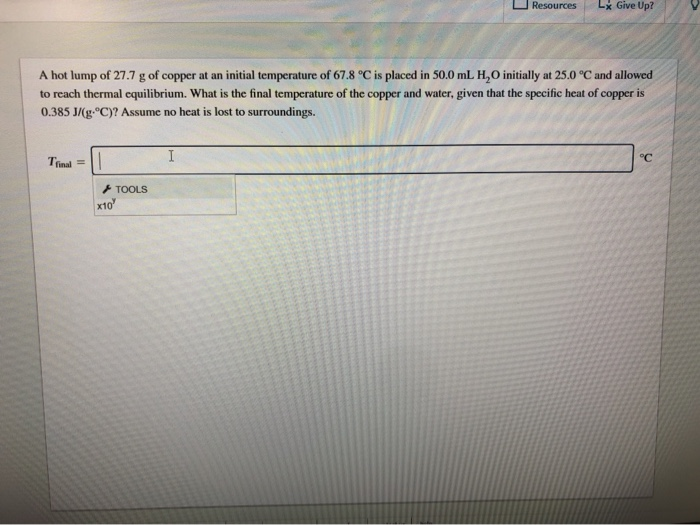Resources Lx Give Up? A hot lump of 27.7 g of copper at an initial temperature of 67.8 °C is placed in 50.0 mL H,O initially at 25.0 °C and allowed to reach thermal equilibrium. What is the final temperature of the copper and water, given that the specific heat of copper is 0.385J/(g.°C)? Assume no heat is lost to surroundings. Trinal = TOOLS *10'

• ### The pressure inside a gas bottle is 3290 kPa when the temperature is 26.0 °C. What...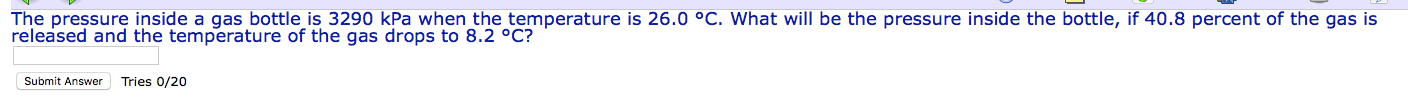The pressure inside a gas bottle is 3290 kPa when the temperature is 26.0 °C. What will be the pressure inside the bottle, if 40.8 percent of the gas is released and the temperature of the gas drops to 8.2 °C? Submit Answer Tries 0/20

• ### Find the vapor pressure, kPa, at a temperature of 90 C Given: • Compound = nOctane...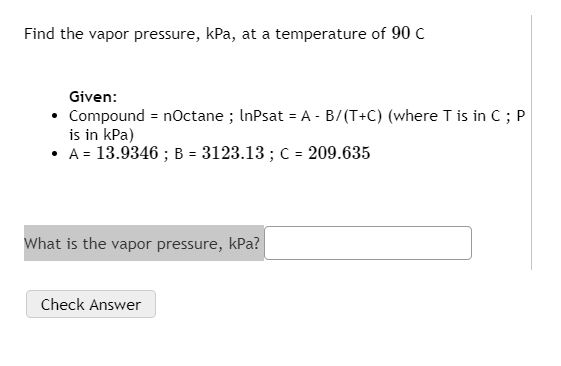Find the vapor pressure, kPa, at a temperature of 90 C Given: • Compound = nOctane ; InPsat = A - B/(T+C) (where T is in C;P is in kPa) • A = 13.9346 ; B = 3123.13 ; C = 209.635 What is the vapor pressure, kPa? Check Answer

• ### 2. Air enters an adiabatic nozzle with a pressure, temperature, and velocity of 900 kPa 500°C, an...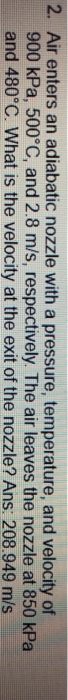2. Air enters an adiabatic nozzle with a pressure, temperature, and velocity of 900 kPa 500°C, and 2.8 m/s, respectively. The air leaves the nozzle at 850 kPa and 480°C. What is the velocity at the exit of the nozzle? Ans 208.949 m/s 2. Air enters an adiabatic nozzle with a pressure, temperature, and velocity of 900 kPa 500°C, and 2.8 m/s, respectively. The air leaves the nozzle at 850 kPa and 480°C. What is the velocity at the exit...

• ### What is the air pressure (kPa) inside the tank? L1=25 cm, L2=35 cm. Water Air u...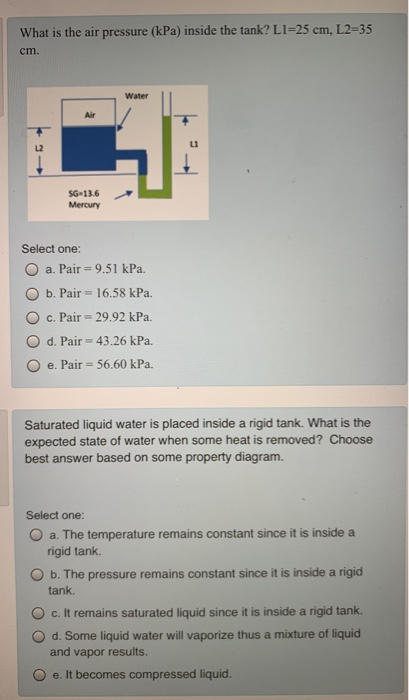What is the air pressure (kPa) inside the tank? L1=25 cm, L2=35 cm. Water Air u SG 13.6 Mercury Select one: a. Pair = 9.51 kPa. b. Pair = 16.58 kPa. c. Pair = 29.92 kPa. d. Pair = 43.26 kPa. e. Pair = 56.60 kPa. Saturated liquid water is placed inside a rigid tank. What is the expected state of water when some heat is removed? Choose best answer based on some property diagram. Select one: O a. The...

• ### 2. The vapor pressure, p, of nitric acid varies with temperature as follows: t/°C 0 20...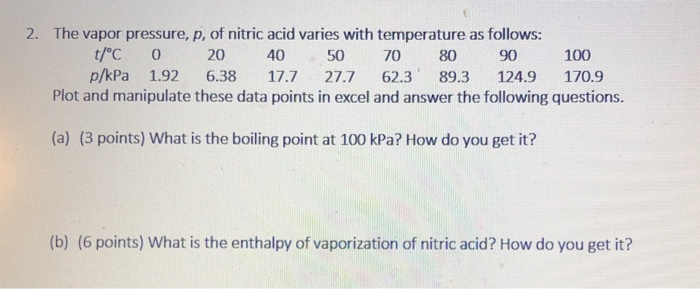2. The vapor pressure, p, of nitric acid varies with temperature as follows: t/°C 0 20 40 50 70 80 90 100 p/kPa 1.92 6.38 17.7 27.7 62.3 89.3 124.9 170.9 Plot and manipulate these data points in excel and answer the following questions. (a) (3 points) What is the boiling point at 100 kPa? How do you get it? (b) (6 points) What is the enthalpy of vaporization of nitric acid? How do you get it?

• ### One of the calculations you will perform using the LabQuest is taking into account the increase in air pressure ins...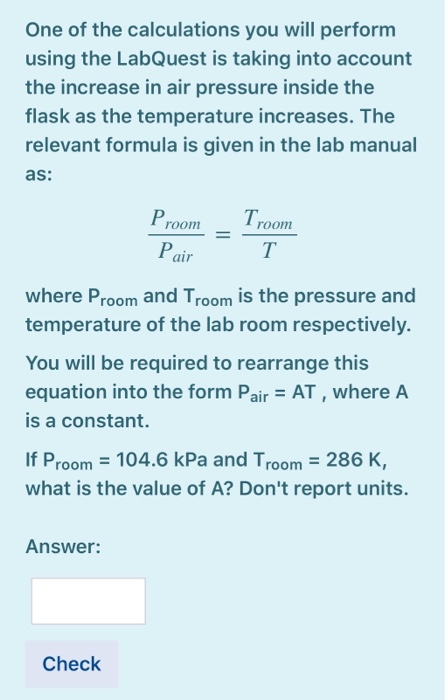One of the calculations you will perform using the LabQuest is taking into account the increase in air pressure inside the flask as the temperature increases. The relevant formula is given in the lab manual as: P room I room Pair where Proom and Troom is the pressure and temperature of the lab room respectively. You will be required to rearrange this equation into the form Pair = AT, where A is a constant. If Proom = 104.6 kPa and...

• ### Air enters an adiabatic nozzle at 500 kPa and a temperature of 200 °C with a...

Air enters an adiabatic nozzle at 500 kPa and a temperature of 200 °C with a velocity of 100 m/s. It exits the nozzle at a pressure of 100 kPa. Assuming that the expansion through the nozzle occurs reversibly, determine (a) the exit temperature and (b) the exit velocity of the air. The specific heats of air can be assumed to be constant with Cv = 0.742 kJ/kg oC and Cp = 1.029 kJ/kg oC.

• ### Air enters an adiabatic nozzle under the following conditions: pressure = 900 kPa temperature = 560°C...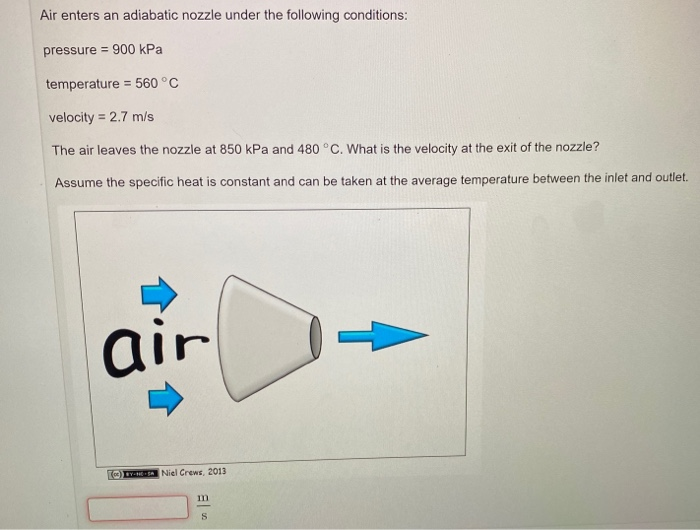Air enters an adiabatic nozzle under the following conditions: pressure = 900 kPa temperature = 560°C velocity = 2.7 m/s The air leaves the nozzle at 850 kPa and 480 °C. What is the velocity at the exit of the nozzle? Assume the specific heat is constant and can be taken at the average temperature between the inlet and outlet. air (c) EYES Niel Crews, 2013